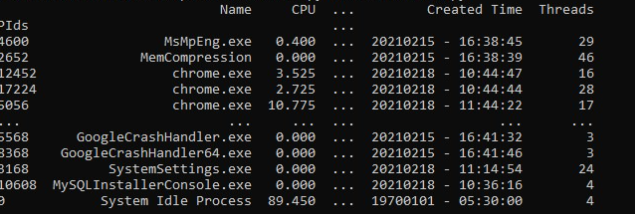# Python: Create an Application Process Monitor Using psutil

In this tutorial, we will use some steps to create an application process monitor using python psutil.1.Import some libraries

```import psutil           #pip install psutil
import datetime
import pandas as pd     #pip install pandas```

2.Create some variables to save information

```pids = []
name = []
cpu_usage= []
memory_usage = []
memory_usage_percentage =[]
status =[]
create_time =[]

4.Get application process information using psutil

```for process in psutil.process_iter():
pids.append(process.pid)
name.append(process.name())

cpu_usage.append(process.cpu_percent(interval=1)/psutil.cpu_count())

memory_usage_percentage.append(round(process.memory_percent(),2))

create_time.append(datetime.datetime.fromtimestamp(process.create_time()).strftime("%Y%m%d - %H:%M:%S"))

status.append(process.status())

You should notice:

• pid(): the process id number
• name(): the name of the process
• cpu_percent(): the percentage of CPU utilization of the process
• memory_info(): memory usage by the process
• memory_percent(): the process memory percentage by comparing the process memory
• create_time(): the process creation time in seconds.
• status(): the running status of the process.
• append(): add the return value to the list.
• round(): sound up the decimal pint number up to 2 digits.
• fromtimestamp(): convert the creation time seconds in readable time format
• strftime() function will convert the date-time object to a readable string

5.Save process information in a python dict

```data = {"PIds":pids,
"Name": name,
"CPU":cpu_usage,
"Memory Usages(MB)":memory_usage,
"Memory Percentage(%)": memory_usage_percentage,
"Status": status,
"Created Time": create_time,
}```

6.Format and output application process information

```process_df = pd.DataFrame(data)
#set index to pids
process_df =process_df.set_index("PIds")

#sort the process
process_df =process_df.sort_values(by='Memory Usages(MB)', ascending=False)

#add MB at the end of memory
process_df["Memory Usages(MB)"] = process_df["Memory Usages(MB)"].astype(str) + " MB"

print(process_df)```

Run this code, you may get this information: